# Differential radio map-based robust indoor localization

## Introduction

Ubiquitous computing and communication have become popular with the development of wireless communication technology over the last decade. The need for location information to capture contexts and configure them into the computing and communication processes, coupled with the unavailability of global positioning system (GPS) in indoor environment, has triggered increased research interest in indoor localization. Recently, numerous localization systems have been developed based on the received signal strength (RSS) of the wireless local area networks (WLANs). The advantage of these systems is that the cost of deploying a specialized infrastructure is avoided. However, building an indoor localization system based on WLAN is a challenging problem due to the complex indoor signal propagation character and different hardware solutions of different mobile devices.

In this article, we propose a novel robust indoor localization algorithm under the framework of Bayesian filter. The particle filter (PF) [12, 13] is adopted to achieve the localization and tracking task, which makes full use of the history observation to improve the estimation accuracy. The differential radio map is used for building the observation likelihood for the PF algorithm so as to solve the dynamic environment and different receiver gain problem. For the sake of predicting signal strength measurements at arbitrary locations so as to calculate particle weight and improve localization accuracy, we also adopt a local linearization technique to realize continuous interpolation of the radio map.

This article is organized as follows. Section II provides a brief overview of the indoor localization problem. In Section III, the definition of the differential radio map is given, and the localization architecture is described from the view of system. The detailed implementation of our differential radio map-based PF algorithm is presented in Section IV. Section V validates our algorithm by evaluations. Finally, the conclusion is drawn in Section VI.

## Related works

Indoor localization with WLAN has been an active research area in the last decade. Honkavirta et al.  presented a survey of location fingerprinting methods, and Seco et al.  reviewed the indoor localization algorithms from the aspect of mathematic. In this section, we give a brief overview of some key research findings in this area. Considering the needs of building the radio map, we divide the methods into map-based and non-map based algorithms.

Besides the above research studies, some schemes based on the deterministic and proba-bilistic framework have also been developed. Lim et al.  used the truncated singular value decomposition to calculate the signal-distance map (SDM) based on the online measurements between the APs. Although the dynamic SDM makes it possible to capture the challenging dynamic indoor environment, the requirements of the high AP density and the modification of commercial AP's software make the scheme impractical. Hossain et al.  proposed a robust localization algorithm that could make use of multiple wireless techniques. Schwaighofer et al.  first adopted the Gaussian process (GP) as a non-parametric tool to realize the approximation of the radio map of cellular network. Ferris et al.  introduced the GP into the WLAN-based indoor localization. Compared with other regression models, the GP takes into account the noise of the observation and provides the ability to approximate nonlinear signal propagation model. Madigan et al.  adopted a Bayesian hierarchical approach to realize indoor localization, which eliminated the need of knowing the locations of the training points. Huang et al.  proposed a similar Bayesian algorithm and introduced the stochastic properties of measurement errors and the reliability of the measurement data into the factor graph framework so as to improve the accuracy. Wymeersch et al.  also proposed a Bayesian localization algorithm and took the cooperation of the nodes into consideration to improve the accuracy. Feng et al.  employed the compressive sensing theory to analyze the localization problem. The localization problem is modeled as a sparse question and can be solved by the L1 minimization. While these methods cut down the measurement effort and partially adapt to the dynamic environment, they still require effort in terms of placing sniffers, modifying commercial AP's software, obtaining the knowledge of AP placement, and of complex computation.

## Differential radio map and system architecture

In this section, we define the differential radio map and analyze its ability to restrain the dynamic environmental noise and receiver gain difference. The architecture of the proposed localization system is presented thereafter.

The construction of the differential radio map is almost the same as that of the traditional radio map, except that a differential operation module is introduced. First, we divide the deployment area into equal square training cells with their vertices set as the training points. Then, we perform off-line training to record RSS measurements transmitted by each AP and store these measurements into the radio map. The radio map has the following form: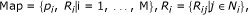(1)

where M is the total number of training points, p i and R i indicate the location and fingerprint of the i th training point, respectively, N i is the detected AP list at the i th training point, R ij is a Gaussian PDF represented by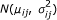with the mean μ ij , and covariance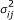, which is used to approximate the RSS measurements collected at the i th training point and transmitted by the j th AP.

Unlike the traditional radio map which adopts the fingerprint R i directly for localization, we adopt the differential fingerprint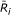which takes the R i as input, and it is calculated as follows: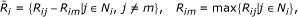(2)

where R im is the strongest RSS measured at the i th training point (the measurement with lower ID is adopted when equal RSSs are measured), and R ij -R im is represented by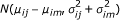.

In theory, the propagation of radio signal is regulated by a certain principle. The shadowing model is widely adopted to approximate the signal propagation character in the indoor circumstance. With this model, R ij is defined by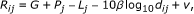(3)

where G is the receiver gain, P j is the transmitting power (dBm) of the j th AP, L j is the signal attenuation power (dBm) at the distance of 1 m, β is the path loss exponent, d ij is the Euclidean distance between the i th training point and j th AP, and v represents the measurement noise. G + P j - L j is always represented by one symbol and is called the received power at the distance of 1 m in other studies. To analyze the feature of the differential operation, we use three symbols to represent each factor here. The dynamic indoor environment always causes the change of parameters L j and β, and the change of these parameters incur the change of the R ij. As for different types of device, the receiver gain G is different, and thus the change of device causes the change of R ij , as well.

The differential RSS is calculated as(4)

where P j - P m is a constant which does not change with environment. While L j and L m change with the environment, they always offset to the same direction. Consequently, the change of L j -L m is not significant compared with the change of L j and L m . As for 10β (log10d ij - log10d im ), since log10d ij - log10d im is less than log10d ij and log10d im , the change must be insignificant, too. From Equation 4, we can see that the term G is eliminated in the expression. Therefore, the differential RSS scheme is immune to the dynamic indoor environment, and can solve the problem caused by different receiver gains of multi-devices.

To validate the above theoretical analysis, we placed two different types of mobile devices at the same location and collected RSS measurements from two APs located at different places. We used an Intel 4965AGN wireless card and a TP-LINK TL-WN322G wireless card to gather RSS. There is a door between the wireless cards and APs, and we closed the door in the time instant 15-45. We define Int1 and Int2 as the RSS measurements, respectively, from AP1 and AP2 by 4965AGN, TP1 and TP2 as the RSS measurements by TL-WN322G, Int_dif as the differential signal of Int1 and Int2, and TP_dif as the differential signal of TP1 and TP2. Figure 1 demonstrates that the change of the differential RSS is quite insignificant compared with the change of RSS. It can also be seen that while the difference of the RSS measurements from the same AP with different devices is remarkable, the difference of differential RSS measurements is almost the same. The average differences between Int1 and TP1, Int2 and TP2 are 10.5 and 11.3, respectively, while that between Int_dif and TP_dif is only 0.8.

### System architecture

The system flow of the proposed differential radio map-based localization system is plotted in Figure 2, where x t is the current estimated location information, y t is the current observation, p (xt- 1|yt- 1) is the posterior PDF estimation of time instant t - 1, p (x t |yt- 1) and p (x t |y t ) are the prior and posterior PDF estimations of time instant t, respectively.

The localization system follows the framework of Bayesian filter [12, 13] and estimates the posterior PDF of the location recursively conditioned on all the measurements, y1,...,y t . The essential of the Bayesian filter is the prediction process and observation update process, which are defined as follows:(5)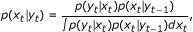(6)

where the term p (x t |xt-1) represents the motion model, which describes the motion of the mobile device, and p (y t |x t ) represents the observation likelihood model, which describes the likelihood of observing a differential RSS measurement vector y t at a location x t . We describe the detailed models in the next section.

## Implementation of the algorithm

This section first gives a brief introduction of the PF algorithm, and then defines the motion model and observation model in detail.

### PF algorithm for localization and tracking

Bayesian filter algorithms  have achieved great success in handling the localization and tracking problem through the sequential estimation of target's state, cooperation with the movement modeling, prior distribution prediction, and posterior distribution estimation. Among these Bayesian filter algorithms, the PF algorithm [12, 13] has emerged as a popular choice because of its unique ability in dealing with the complex non-Linear and non-Gaussian estimation problem. For these reasons, we adopt the PF algorithm for localization in this article. The PF algorithm approximates the probability distribution of the estimated target with several weighted particles, and deals with the sequential estimation by carrying out a series of particle-propagating operations. Let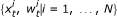denote a particle set, where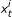is a sample of x t with associated normalized weight. Then, the posterior PDF p (x t |y t ) can be approximated as(7)

where δ () is the Dirac delta function. Suppose we have the proposal distribution q (x t |xt-1, y t ), then the predicted locationcan be generated based on the previous location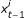and the latest observation y t as follows: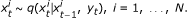(8)

andis given by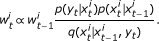(9)

Most articles in the literature define the proposal distribution as q (x t |xt-1, y t ) = p (x t |xt-1), which only considers the motion model and neglects the latest observation y t . For simplicity, we follow this scheme. Interested readers could find out the methods of designing advanced proposal distribution in . Now, the question is how to define the motion modeland the observation likelihood model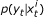for the particles.

### Motion model

Speaking in general, the motion model of the mobile devices in the indoor environment is untraceable, and the velocity and direction of the mobile device are hard to estimate. In , Ferris et al. used a voronoi graph to aid the motion prediction. Although the performance of the prediction is improved, it needs detailed floor plan of the building which is impractical and inconvenient. Jin et al.  adopted dead reckoning sensors to make prediction, which needs the additional expensive sensors. Based on the principle of making the system as universal as possible, we define the motion model purely based on the historical motion information. We consider only two-dimensional localization, and the extension to the three-dimensional scenario is straightforward. The two-dimensional location state x t can be represented by (X t , Y t ). Suppose the location states of the previous two time instants are, xt-2and xt-1, the predicted velocityand direction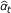of the mobile device can be estimated as follows: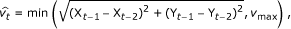(10)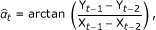(11)

where vmax is the velocity threshold of the mobile device and generally set as 1m/s. The motion model of the particles can be written as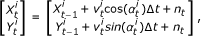(12)

where n t is the noise,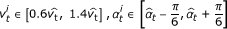. The new particles are generated in the fan-shaped area. Meanwhile, to cope with the circumstance such as the swerve of the mobile device, we randomly select 80% particles that participate in the prediction defined by Equation 12, and let the rest 20% particles randomly move in the circular area, with the last estimation as center and vmax as radius.

### Observation likelihood model

In order to calculate the likelihood of the particles at arbitrary locations, the first thing that should be solved is to fulfill the radio map with the ability of representing the RSS measurements at arbitrary locations. The radio map defined by Equation 1 is a discrete map, and only the RSS fingerprints of the training points are recorded. We adopt a linearization technique to predict RSS measurements at arbitrary locations. Within each of the square training cells, we use a hyperplane to approximate the RSS measurements of every AP. The 3D plane equation includes (x, y, r) coordinate, where (x, y) is the 2D location, and r is RSS measurement. The equation is defined as(13)

where a x , a y , and a r are the coefficients of the plane equation, and c is a non-zero constant and set as 1 in this article. The RSS measurements of the four vertices and their locations are known, therefore, they can be used to calculate the coefficients of the hyperplane equation. Suppose the locations of the four vertices are (x1, y1), (x2, y2), (x3, y3), and (x4,y4), the mean values of the RSS measurements from one AP are r1, r2, r3, and r4. We have a constraint matrix in the following form: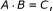(14)

where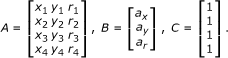(15)

The coefficients of the hyperplane equation can be solved with the least square algorithm as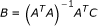, where ()-1 and ()T represent the matrix inverse and transpose, respectively. After building the hyperplane equation for every AP in each of the square training cells, the mean value of RSS fingerprint at an arbitrary location can be calculated with Equation 13, and the average value of the four vertices' covariance is set as the covariance of the location.

For the particle, we first judge which training cell it belongs to and calculate the RSS fingerprint vector based on the hyperplane equation, and then, we transform the RSS fingerprint vector and the current, observed RSS measurement vector to their differential form. Suppose the differential fingerprint for the j th AP is, the observed differential RSS measurement from the j th AP is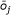, then the likelihood for the j th AP is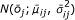. Suppose the RSS measurements from different APs are independent, the observation likelihood can be expressed as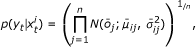(16)

where n is the number of detected APs. The exponent 1/n is used to smooth the likelihood so as to avoid the occurring of overconfident estimate. We calculate the observation likelihood values for all the particles and adopt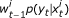as the new particle weight, and then, normalize it to ensure the summation of weights is equal to 1.

With the normalized weighted particle set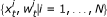, the location estimation x t can be calculated as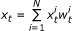. To avoid the occurrence of degeneration problem, we adopt the adaptive systematic resample method  for further optimizing the quality of the particles. The outline of our differential radio map-based Bayesian localization (DRMBL) algorithm is summarized in Table 1.

## Experimental evaluation

In this section, we start by giving a detailed presentation of our testbed. Then, we evaluate the proposed improvement strategies under different conditions. For simplicity and comparison, the deterministic fingerprint-based RADAR algorithm adopts the weighted k-nearest neighbor technique, abbreviated as KNN, and the algorithm that adopts probabilistic fingerprint localization technique is abbreviated as PL. Furthermore, to evaluate the effectiveness of our differential strategy, the differential strategy is also applied to the KNN and PL algorithms. We define the improved algorithms as DRMKNN and DRMPL, respectively.

### Experiment setup

To evaluate the performance of our algorithm, we performed realistic experiment on the second floor of the Electronic Information building of Dalian University of Technology. The layout of the floor is shown in Figure 3, and a scene of training is illustrated in Figure 4. The deployment area has a dimension of 72 × 29 meters. The TP-LINK TL-WR340G+ APs are adopted, and there are six detectable APs on the floor. We use an IBM ThinkPad X60 laptop with Windows XP operating system as the mobile device. A TP-LINK TL-WN322G wireless card is adopted to gather RSS in the radio map building phase, and a 4965AGN card and a TL-WN322G wireless card are used in the localization phase. Unless otherwise specified, we adopted the data collected by 4965AGN in the following evaluations. We designed the software for collecting RSS based on the NDIS Miniport .

The radio map has 164 locations along the corridor and 70 locations inside the room. We placed the training points 1.2 m apart and collected 10 samples at each point with a time interval of 1 s. The default parameters for the algorithms are as follows: the parameter k of KNN is 4, and the number of particles used in the DRMBL is 1000.

### Evaluation results

Figure 5a demonstrates the comparison of the cumulative distribution function (CDF) of the localization error when using a TL-WN322G wireless card which is the same as that used in the training phase; the DRMBL algorithm outperforms other algorithms and its average localization error decreases by 13% compared with KNN. The average localization errors of DRMKNN and DRMPL algorithms decrease by 4% and 3.7%, respectively, when comparing with KNN and PL algorithms. Figure 5b shows the localization error when using an Intel 4965AGN wireless card. It can be seen that the performances of DRMBL, DRMKNN, and DRMPL algorithms are nearly the same as that of Figure 5a, while the performance of KNN and PL algorithms drops dramatically. These results show the effectiveness of our improvement strategies, and indicate that the differential method is generic and can be applied to other localization algorithms to improve their accuracy.

Table 2 summarizes the detailed results. It can be seen that comparing with KNN and PL algorithms, the average error of DRMBL decreases by more than 26% and 22%, respectively. With our differential strategy, the average errors of DRMKNN and DRMPL decrease by more than 18% and 10%. However, it should be mentioned that the maximum errors of DRMKNN and DRMPL increases, respectively, by 35% and 36%. This is because when the RSS measurements from the reference AP incur from severe noise, the differential operation may bring noise to the differential RSS vector, which incurs the increase of localization error. Hence, the selection of reference AP is of vital importance.

To evaluate the performance of our DRMBL algorithm under different conditions, we studied the sensitivity of the algorithm to the number of APs and radio map spacing. Figure 6a illustrates that the average localization error decreases gradually with the increase of the number of APs, and a relatively stable condition can be achieved when the number of APs is greater than 4. In Figure 6b, the error bars represent the standard deviation. It demonstrates nearly the same character as that of average error. It can be seen from Figure 7 that the accuracy decreases and standard deviation increases gradually with the increase of radio map spacing. However, the DRMBL algorithm still achieves average error of 2.56 m at the radio map spacing of 9.6 m. It can be learned from Figure 7a that the performances of DRMKNN and DRMPL drop dramatically with the increase of radio map spacing; because with the decrease of the number of training points, the RSS measurement from the reference AP may be far away from any training points, which introduces noise to the differential RSS vector.

Essentially speaking, our DRMBL algorithm is a PF algorithm, and the performance of PF algorithm depends largely on the number of particles adopted in the algorithm. We evaluated the algorithm using different number of particles. Figure 8 illustrates that with the increase of the number of particles, the average error and its standard deviation decrease gradually. It can be seen that 100 particles are sufficient for the algorithm to achieve reasonable results.

Figure 9 demonstrates the tracking effect of our DRMBL algorithm. A mobile device is moving in the deployment area, its motion trace covers straight corridor with excellent AP signal coverage and corner with noisy and feeble AP signal. In Figure 9a, the blue "L" shape line is the ground truth trace, and the black trace is the estimated one. We can see that, in the corridor, the tracking performance is very good; however, in the corner, the estimation error increases remarkably. For clarity, we mark the poor estimations with red stars and plot the detailed estimation errors in Figure 9b. From Figure 9, we can learn that one should pay special attention to the placement of APs to ensure that the radio signal could cover every corner in the deployment area.

### Discussion

We highlight some of the experience on the DRMBL algorithm, including the differential strategy, the density of training points, and the characteristic of the PF algorithm.

It should be mentioned that the differential strategy is generic. It can be adopted by any localization system as long as they use the RSS as measurement, such as WLAN, Zigbee, Bluetooth, and GSM-based systems. If N APs are detected, ideally there are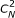pairs of differential RSS measurements. For simplicity, we select the AP with the strongest RSS as the reference AP and select N - 1 differential RSS measurements relative to it according to Equation 2. Computational resources permitting, one can utilize all the differential measurements to improve the performance. The performance of the differential strategy is greatly affected by the reference AP. Therefore, the selection of the most appropriate reference AP is vital for the DRMBL algorithm. We have verified four criteria: AP with the strongest RSS, AP with the weakest RSS, AP with the minimum standard deviation, and AP with the maximum standard deviation. Table 3 illustrates that the criterion of selecting the AP with the strongest RSS is superior to others.

As has been seen from Figure 7a, one shortcoming of the differential strategy is that it requires dense training points. Our DRMBL algorithm solves this problem with continuous interpolation technique. When the differential strategy is adopted, we suggest that the interpolation technique could be used for generating some virtual training points. Figure 10 demonstrates the results of DRMKNN and DRMPL with interpolation technique used in the radio maps of 4.8 and 9.6 m spacings.

Moreover, with the essential similarity to PF algorithm, DRMBL deals with the location estimation problem by means of prediction and observation update process. In this article, we suppose that the algorithm knows nothing about the prior knowledge; if we have some prior information about the velocity or direction, the tracking accuracy will be improved significantly. Figure 11 demonstrates the localization error for tracking the same trace as that of Figure 9a while having prior velocity information. The estimation error is remarkably reduced, and the average localization error is only 1.17 m.

## Conclusion

To address the problem of realizing accurate localization in complex dynamic environment in the WLAN which is made up of different types of devices, we proposed a novel DRMBL algorithm under the Bayesian framework. We proposed a differential strategy to overcome the common mode noise and a continuous interpolation technique to accurately realize the particle weight calculation. The experiments validated our proposed schemes, and revealed that DRMBL algorithm could achieve reasonable localization results under challenging background conditions. The schemes proposed in this article are generic and could be adopted by other localization systems.

## Abbreviations

CDF:

cumulative distribution function

DRMBL:

GPS:

global positioning system

PDF:

probability distribution function

SDM:

signal-distance map

WLAN:

wireless local area network.

## References

1. Bahl P, Padmanabhan VN: RADAR: an in-building RF-based user location and tracking system. In Proceedings of the 19th IEEE International Conference on Computer Communications (INFOCOM '00). Volume 2. Tel Aviv, Israel; 2000:775-784.

2. Deasy TP, Scanlon WG: Simulation or measurement: the effect of radio map creation on indoor WLAN-based localisation accuracy. Wirel Personal Commun 2007, 42(4):563-573. 10.1007/s11277-006-9211-x

3. Tsai TC, Li CL, Lin TM: Reducing calibration effort for WLAN location and tracking system using segment technique. In Proceedings of the IEEE International Conference on Sensor Networks, Ubiquitous, and Trustworthy Computing (SUTC '06). Volume 2. Taichung, Taiwan; 2006:46-51.

4. Chai XY, Yang Q: Reducing the calibration effort for probabilistic indoor location estimation. IEEE Trans Mobile Comput 2007, 6(6):649-662.

5. Philipp B: Redpin--adaptive, zero-configuration indoor localization through user collaboration. In Proceedings of the 1st ACM international workshop on Mobile entity localization and tracking in GPS-less environments (MELT '08). San Francisco, USA; 2008:55-60.

6. Chintalapudi K, Iyer AP, Padmanabhan VN: Indoor localization without the pain. In Proceedings of the 16th Annual International Conference on Mobile Computing and Networking (MOBICOM '10). Chicago, USA; 2010:173-184.

7. Wu Y, Hu JB, Chen Z: Radio map filter for sensor network indoor localization systems. In Proceedings of the 5th IEEE International Conference on Industrial Informatics (INDIN '07). Vienna, Austria; 2007:63-68.

8. Youssef M, Agrawala A: The Horus location determination system. Wirel Netw 2008, 14(3):357-374. 10.1007/s11276-006-0725-7

9. Chen YC, Chiang JR, Chu HH, Huang P, Tsui AW: Sensor-assisted wi-fi indoor location system for adapting to environmental dynamics. In Proceedings of the 8th ACM international symposium on Modeling, analysis and simulation of wireless and mobile systems (MSWiM '05). Montreal, Canada; 2005:118-125.

10. Yin J, Yang Q, Ni LM: Learning adaptive temporal radio maps for signal-strength-based location estimation. IEEE Trans Mobile Comput 2008, 7(7):869-883.

11. Fang SH, Lin TN: A dynamic system approach for radio location fingerprinting in wireless local area networks. IEEE Trans Commun 2010, 58(4):1020-1025.

12. Doucet A, Godsill S, Andrieu C: On sequential Monte Carlo sampling methods for Bayesian filtering. Stat Comput 2000, 10(3):197-208. 10.1023/A:1008935410038

13. Djuric PM, Kotecha JH, Zhang JQ, Huang YF, Ghirmai T, Bugallo MF, Miguez J: Particle filtering. IEEE Signal Process Mag 2003, 20(5):19-38. 10.1109/MSP.2003.1236770

14. Honkavirta V, Perala T, Ali-Loytty S, Piche R: A comparative survey of WLAN location fingerprinting methods. In Proceedings of the 6th Workshop on Positioning, Navigation and Communication (WPNC '09). Hannover, Germany; 2009:243-251.

15. Seco F, Jimenez AR, Prieto C, Roa J, Koutsou K: A survey of mathematical methods for indoor localization. In Proceedings of the 6th IEEE International Symposium on Intelligent Signal Processing (WISP '09). Budapest, Hungary; 2009:9-14.

16. Lim H, Kung LC, Hou JC, Luo HY: Zero-configuration, robust indoor localization: theory and experimentation. In Proceedings of the 25th IEEE International Conference on Computer Communications (INFOCOM '06). Barcelona, Spain; 2006:1-12.

17. Hossain M, Van HN, Jin Y, Soh WS: Indoor localization using multiple wireless technologies. In Proceedings of the 4th IEEE International Conference on Mobile Adhoc and Sensor Systems (MASS '07). Pisa, Italy; 2006:1-8.

18. Schwaighofer A, Grigoras M, Tresp V, Hoffmann C: GPPS: a Gaussian process positioning system for cellular networks. In Proceedings of the 7th Conference on Neural Information Processing Systems (NIPS '03). Whistler, Canada; 2003:1-8.

19. Ferris B, Hahnel D, Fox D: Gaussian processes for signal strength-based location estimation. In Proceedings of the 2nd Robotics: Science and Systems (RSS '06). Philadelphia, USA; 2006:1-8.

20. Madigan D, Einahrawy E, Martin RP, Ju WH, Krishnan P, Krishnakumar AS: Bayesian indoor positioning systems. In Proceedings of the 24th IEEE International Conference on Computer Communications (INFOCOM '05). Volume 2. Miami, USA; 2005:1217-1227.

21. Huang CT, Wu CH, Lee YN, Chen JT: A novel indoor RSS-based position location algorithm using factor graphs. IEEE Trans Wirel Commun 2009, 8(6):3050-3058.

22. Wymeersch H, Lien J: MZ Win, Cooperative localization in wireless networks. Proc IEEE 2009, 97(2):427-450.

23. Feng C, Au WSA, Valaee S, Tan ZH: Compressive sensing based positioning using RSS of WLAN access points. In Proceedings of the 29th IEEE International Conference on Computer Communications (INFOCOM '10). San Diego, USA; 2010:1631-1639.

24. Fox D, Hightower J, Lin L, Schulz D, Borriello G: Bayesian filtering for location estimation. IEEE Pervas Comput 2003, 2(3):24-33. 10.1109/MPRV.2003.1228524

25. Johansen AM, Doucet A: A note on auxiliary particle filters. Stat Probab Lett 2008, 78(12):1498-1504. 10.1016/j.spl.2008.01.032

26. Jin YY, Motani M, Soh WS, Zhang JJ: SparseTrack: enhancing indoor pedestrian tracking with sparse infrastructure support. In Proceedings of the 29th IEEE International Conference on Computer Communications (INFOCOM '10). San Diego, USA; 2010:668-676.

27. The Code Project[http://www.codeproject.com/KB/IP/wlanscan_ndis.aspx]

## Acknowledgements

This study was supported by the National Natural Science Foundation of China under grant numbers 60871046 and the National Hi_Tech Research and Development 863 program of China under grant numbers 2008AA092701. The authors thank the constructive discussions with their group members during the writing of the article. The authors would also like to thank the reviewers for their useful suggestions and comments.

## Author information

Authors

### Corresponding author

Correspondence to Jie Wang.

### Competing interests

The authors declare that they have no competing interests.

## Authors’ original submitted files for images

Below are the links to the authors’ original submitted files for images.

## Rights and permissions

Reprints and Permissions

Wang, J., Gao, Q., Wang, H. et al. Differential radio map-based robust indoor localization. J Wireless Com Network 2011, 17 (2011). https://doi.org/10.1186/1687-1499-2011-17

• Accepted:

• Published:

• DOI: https://doi.org/10.1186/1687-1499-2011-17

### Keywords

• Indoor localization